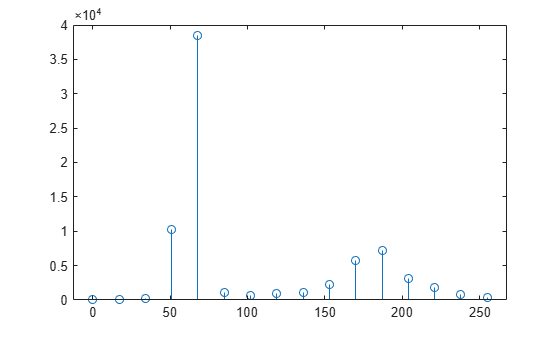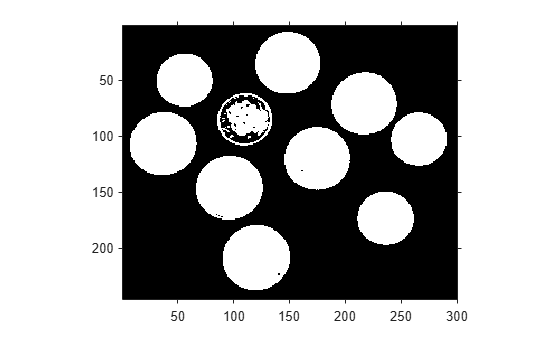otsuthresh

Global histogram threshold using Otsu's method

Description

example

T = otsuthresh(counts) computes a global threshold T from histogram counts, counts, using Otsu's method . Otsu's method chooses a threshold that minimizes the intraclass variance of the thresholded black and white pixels. The global threshold T can be used with imbinarize to convert a grayscale image to a binary image.

[T,EM] = otsuthresh(counts) returns the effectiveness metric, EM, which indicates the effectiveness of the thresholding.

Examples

collapse all

Calculate a 16-bin histogram for the image.

[counts,x] = imhist(I,16);
stem(x,counts)Compute a global threshold using the histogram counts.

T = otsuthresh(counts);

Create a binary image using the computed threshold and display the image.

BW = imbinarize(I,T);
figure
imshow(BW)Input Arguments

collapse all

Histogram counts, specified as a vector of nonnegative numbers.

Data Types: single | double | int8 | int16 | int32 | int64 | uint8 | uint16 | uint32 | uint64

Output Arguments

collapse all

Global threshold, returned as a numeric scalar in the range [0, 1].

Data Types: double

Effectiveness metric of the threshold, returned as a numeric scalar in the range [0, 1]. The lower bound is attainable only by histogram counts with all data in a single non-zero bin. The upper bound is attainable only by histogram counts with two non-zero bins.

Data Types: double

 Otsu, N., "A Threshold Selection Method from Gray-Level Histograms." IEEE Transactions on Systems, Man, and Cybernetics. Vol. 9, No. 1, 1979, pp. 62–66.